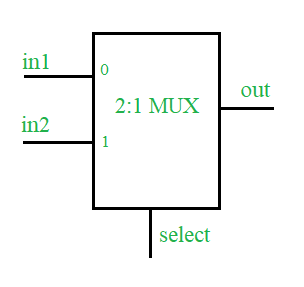# Multiplexer Design using Verilog HDL

• Difficulty Level : Hard
• Last Updated : 07 Jun, 2021

Prerequisite – Multiplexers in Digital Logic
Problem :
Design of a 2:1 MUX using Verilog Hardware Description Language along with Testbench.

Concepts :
A multiplexer is a combinational type of digital circuits that are used to transfer one of the available input lines to the single output and, which input has to be transferred to the output it will be decided by the state(logic 0 or logic 1) of the select line signal. 2:1 Multiplexer is having two inputs, one select line (to select one of the two input) and a single output.Truth Table –

Verilog HDL code of 2:1 MUX :

Design –

```// define a module for the design
module mux2_1(in1, in2, select, out);

// define input  port
input in1, in2, select;

// define the output port
output out;

// assign one of the inputs to the output based upon select line input
assign out = select ? in2 : in1;
endmodule :mux2_1```

Testbench –

```module test;
reg in1, in2, select;
wire out;

// design under test
mux2_1 mux(.in1(in1), .in2(in2),
.select(select), .out(out));

// list the input to the design
initial begin in1=1'b0;in2=1'b0;select=1'b0;
#2    in1=1'b1;
#2    select=1'b1;
#2    in2=1'b1;
#2    \$stop();
end

// monitor the output whenever any of the input changes
initial begin \$monitor("time=%0d, input1=%b, input2=%b,
select line=%b, output=%b", \$time,
in1, in2, select, out);
end
endmodule :test```

Expected Output –

```time=0, input1=0, input2=0, select line=0, out=0
time=2, input1=1, input2=0, select line=0, out=1
time=4, input1=1, input2=0, select line=1, out=0
time=6, input1=1, input2=1, select line=1, out=1 ```
My Personal Notes arrow_drop_up